## TyroCity

Physics XI Notes for Physics Notes

Posted on • Updated on

# Law Of Conservation Of Angular Momentum

The law of conservation of angular momentum states that:
“When the net external torque acting on a system about a given axis is zero, the total angular momentum of the system about that axis remains constant.”

Mathematically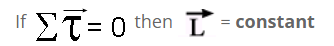Proof

According to the second law of motion net force acting on a body is equal to its rate of change of linear momentum.
i.e.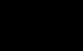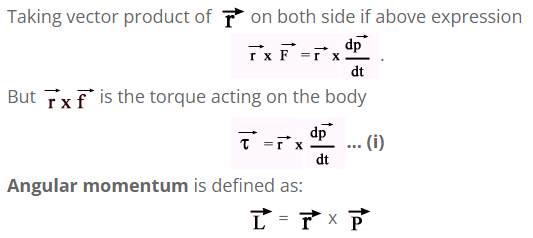Differentiating both sides with respect to "t"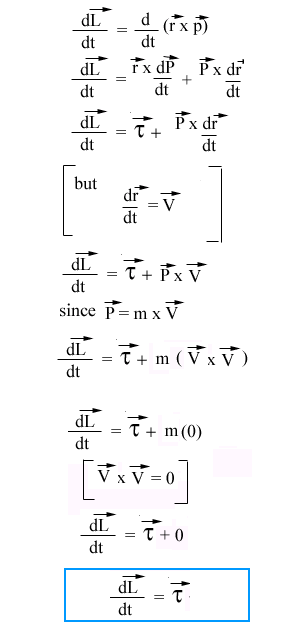Which is the required equation.
This expression states that the torque acting on a particle is the time rate of change of its angular momentum. If the net external torque on the particle is zero, then,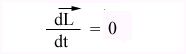Or,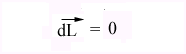Thus the angular momentum of a particle is conserved if and only if the net external torque acting on a particle is zero.